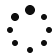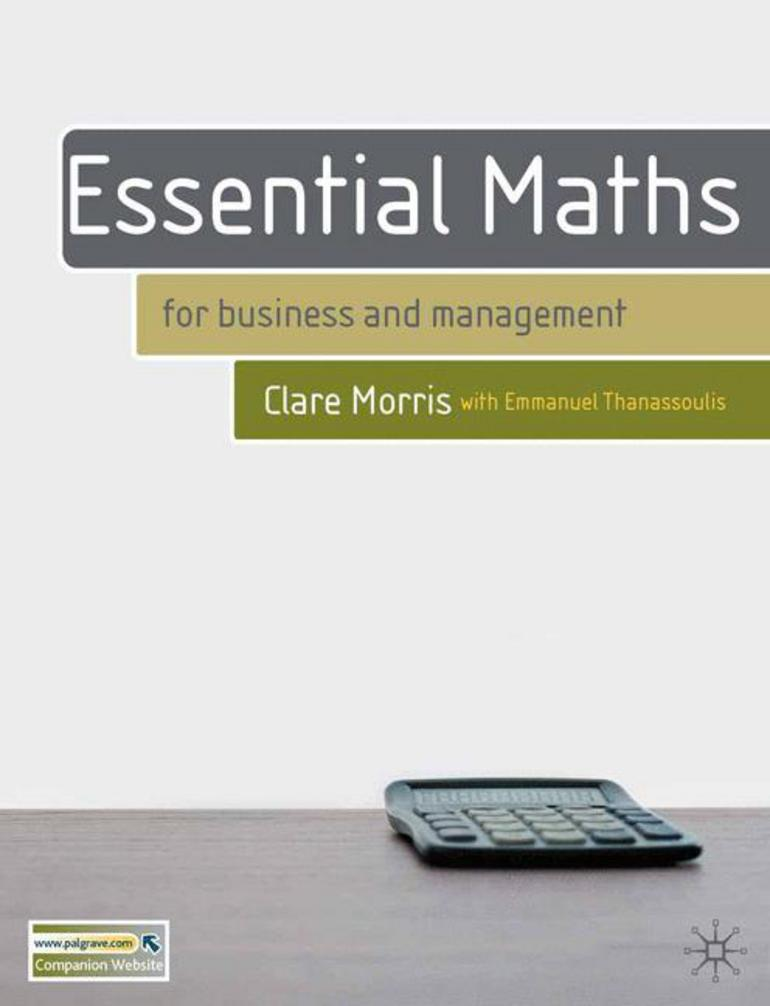## Learning Objectives

• understand why anyone aiming at a business career needs to be reasonably proficient in certain areas of mathematics
• have had a chance to reflect on your previous experiences of studying mathematics, and on the strengths and weaknesses of your personal learning style
• be aware of the use and limitations of spreadsheets and calculators when carrying out mathematical processes.

Back to the top of the page

### Chapter 2 - Arithmetic – working with numbers

• be able confidently to carry out arithmetic operations involving positive and negative numbers, fractions, decimals and percentages
• have developed your ability to estimate roughly what the result of a calculation should be
• know how to round numbers to a given number of decimal places or significant figures, and be able to make a sensible decision as to how many figures to quote in a practical context
• be aware of a range of symbols for mathematical operations and understand their meanings
• be comfortable in reading and writing arithmetical expressions, and understand the technical terminology which is used in this context.

Back to the top of the page

### Chapter 3 - Introducing algebra

• be able to explain why algebra can be a useful tool in a business context
• be confident in manipulating algebraic expressions involving powers, roots, brackets and arithmetical operations
• be able to express simple practical problems in algebraic terms
• be familiar with the terminology used to interpret algebraic expressions.

Back to the top of the page

### Chapter 4 - Graphs

• appreciate the reasons for using graphical methods
• be able to plot graphs using numerical information
• be able to plot graphs of simple algebraic relationships
• be able to interpret information presented graphically
• know how to recognise a linear equation and to interpret the numerical constants in such an equation
• be aware of some important types of non-linear relationship.

Back to the top of the page

### Chapter 5 - Solving equations

• understand what is meant by an equation and its solution
• be able to recognise and solve linear equations
• be able to recognise and solve quadratic equations using the formula method
• be able to solve two simultaneous linear equations
• feel confident in manipulating formulae
• understand inequalities and be able to manipulate them correctly
• be able to build equations to represent practical situations, and to interpret their solutions appropriately.

Back to the top of the page

### Chapter 6 - Introducing calculus

• be able to define what is meant by the slope of a curve at a point
• know what is meant by differentiation, derivative and rate of change
• be able to find the derivative of algebraic expressions involving powers of x
• understand the significance of second derivatives, and be able to use them to find maximum/minimum values of a function
• be able to apply the processes of calculus to the solution of practical problems.

Back to the top of the page

### Chapter 7 - Solving practical problems with mathematics

• be able to translate practical problems expressed verbally into a mathematical format, to use mathematical ideas to solve the problem, and to interpret your solution in the practical terms of the original problem
• be aware of the simplifications which may take place in making the transition from a practical to a mathematical format
• be aware of the potential limitations on the extent to which the mathematical solution may be practically useful.

Back to the top of the page

### Chapter 8 - Statistics: organising data

• be able to describe and give examples of different types of statistical data
• understand the methods by which statistical data may be obtained
• be able to organise data into suitable tables
• know how to construct a range of charts to represent data, and be able to make an appropriate choice of chart for a specific set of data
• be confident in understanding and if necessary criticising published tables and charts.

Back to the top of the page

### Chapter 9 - Statistics: summarising data

• understand why it is desirable to calculate summary measures for a set of data, and what features of the data these measures might represent
• be able to define the mean, median and mode of a set of data, and to calculate these by hand in simple cases
• be able to define the standard deviation, quartiles and mode of a set of data, and to calculate these by hand in simple cases
• be able to interpret the summary measures in practical terms, and to make an informed choice of the best measure to use in a given situation
• know what is meant by skewness, and be able to describe in qualitative terms the skewness or symmetry of a distribution.

Back to the top of the page.